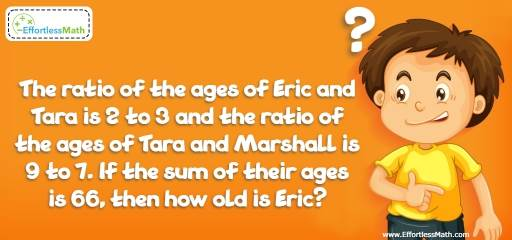# Algebra Puzzle – Challenge 35

Want to improve your logic and critical thinking? Have a look at this great math puzzle. Let's tease your brain!## Challenge:

The ratio of the ages of Eric and Tara is 2 to 3 and the ratio of the ages of Tara and Marshall is 9 to 7. If the sum of their ages is 66, then how old is Eric?

### The Absolute Best Book to challenge your Smart Student!

Let E be the age of Eric, T be the age of Tara and M be the age of Marshall.
E + T + M = 66
The ratio of E to T is 2 to 3. So:
$$E = \frac{2}{3} T$$
The ratio of T to M is 9 to 7. So:
$$M = \frac{7}{9} T$$
Replace the value of E and M in the first equation with their values in the second and third equations:
$$\frac{2}{3} T + T + \frac{7}{9} T = 66 → \frac{22}{9} T = 66 → T = 27$$
$$E = \frac{2}{3} T ⇒ E = \frac{2}{3} (27) = 18$$
Eric is 18 years old now.

### What people say about "Algebra Puzzle – Challenge 35 - Effortless Math: We Help Students Learn to LOVE Mathematics"?

No one replied yet.

X
30% OFF

Limited time only!

Save Over 30%

SAVE $5 It was$16.99 now it is \$11.99